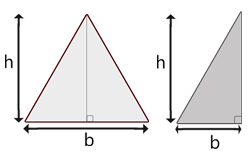# Calculate the area of a triangle

Calculate the area of a right-angled triangle. It is the same formula in both types of triangles in the figure. The mark b in the figure is however a bit misleading since b in one figure is not the same distance as b in the other.#### What is broken?

Is there something wrong with the calculation Calculate the area of a triangle? Is it a bug or has it gone completely offline? Please, let us know what is wrong! If the calculation did not give you the result you expected, please write which values you used and what you expected the calculation to do.

#### Bugs & Fixes

This calculation was last reviewed or fixed: 2011/09/29

Bug / Fix When Name Comment